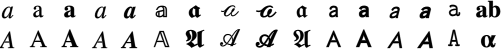prev ( testsuite > Presentation > TokenElements > mi > mimathvariant13  ) next

Sample Rendering:$\begin{array}{cccccccccccccccc}a& \mathrm{a}& \mathbf{a}& \mathit{a}& \mathbit{a}& \mathbb{a}& {a}& \mathcal{a}& \mathsc{a}& \mathfrak{a}& \mathsf{a}& \mathbsf{a}& \mathsfit{a}& \mathbsfit{a}& \mathtt{a}& \mathbf{ab}\\ A& \mathrm{A}& \mathbf{A}& \mathit{A}& \mathbit{A}& \mathbb{A}& {A}& \mathcal{A}& \mathsc{A}& \mathfrak{A}& \mathsf{A}& \mathbsf{A}& \mathsfit{A}& \mathbsfit{A}& \mathtt{A}& \mathbf{\alpha }\end{array}$